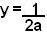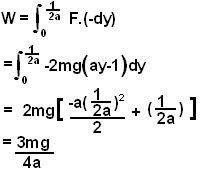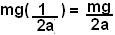Friday, May 2, 2008

Irodov Problem 1.130Frankly the wording of the problem confused me a bit initially since the positive direction of F was not clarified in the problem. However application of some common sense will tell us that F is considered to be in the same direction as that of gravity. It is evident from the problem that the value of F when y=0 i.e. the mass is at the ground, is -2mg. At this point the mass is supposed to accelerate upwards. This can only happen if -2mg means 2mg force directed upwards. In other words the positive direction of F must be aligned with the direction of gravity.

At any given time, there are two forces acting on the mass, the force of gravity mg and the force F, so that the total force acting on the mass is F+mg. This means that when the body starts its motion upwards, there is a net force of mg - 2mg = -mg (acting upwards). As the body moves upwards and its height y increases the magnitude of F keeps decreasing. At a heightthe magnitude of the force F becomes zero. After this point, F reverses its direction and starts to act in the same direction as the force of gravity. The action of the force is completely symmetric (like a mirror image) about the height as shown in the figure. In other words if you take any two heightsand, the force F at these heights will be equal and opposite. From this symmetry it is evident that the maximum height achieved h will be. This is indicated in the figure.

The work done by force F during the first half of the accent will be positive since the direction of motion (up) is same as the direction of the force (up). This can be evaluated as,The reason we use the negative sign in (-dy) is since the direction of increase of dy is in the upwards direction whereas we have chosen the positive direction to be downwards for F.

The gain in potential energy is simply given bysince at the end of half its accent its at a height of 1/2a.